# Lagged correlation

Convolution or cross-correlation are similar operands that can be applied to any square-integrable real functions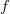$f$ and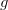$g$.

## Convolution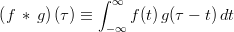$\left( f \, * \, g \right)(\tau) \equiv \int_{-\infty}^{\infty} f(t) \, g(\tau-t) \,dt$.

## Cross-correlation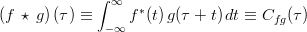$\left( f \, \star \, g \right)(\tau) \equiv \int_{-\infty}^{\infty} f^{*}(t) \, g(\tau+t) \,dt \equiv C_{fg}(\tau)$.
In particular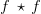$f \, \star \, f$ is designated the autocorrelation.

### Normalized

$C}_{fg}(\tau) \equiv \frac{\int_{-\infty}^{\infty} F^{*}(t) \, G(\tau+t) \,dt}{\int_{-\infty}^{\infty} F^{*}(t) \, F(t) \,dt \, \int_{-\infty}^{\infty} G^{*}(t) \, G(t) \,dt} = \left< \frac{F(t)}{\|F\|} ,\, \frac{G(\tau+t)}{\|G\|$ , where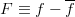$F \equiv f - \overline{f}$. The idea is to remove the average and, optionally, other linear or physical trends from$f$.
In particular we have
$C}_{fg}(0) = \left< \frac{F}{\|F\|} ,\, \frac{G}{\|G\|$ .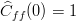$\widehat{C}_{ff}(0) = 1$.

### Discretized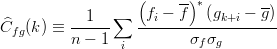$\widehat{C}_{fg}(k) \equiv \frac{1}{n-1} \sum_{i} \frac{ \left( f_i - \overline{f} \right)^{*} \left( g_{k+i} - \overline{g} \right) }{\sigma_f \sigma_g}$, where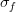$\sigma_f$ is the variance of$f$.
In particular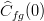$\widehat{C}_{fg}(0)$ is the famous correlation coefficient.

## Example

When both$f$ and$g$ are even functions then the convolution and cross-correlation products are rigorously equivalent. Below, we show these products when applied to box functions (left figure) and to gaussian functions (right figure). In both cases, they are even functions.$f$ is represented by the red line,$g$ is represented by the blue line, the product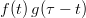$f(t) \, g(\tau - t)$ is represented by the solid blue line. The solid blue area is the convolution product for lag-time$\tau$ and the whole convolution product function (for all$\tau$) is represented by the green line.

The big conclusions we can make is that if$f$ and$g$ are the same functions, but with a lag of$\epsilon$ between them, then the cross-correlation product is maximum when$\tau = \epsilon$.

## Matlab functions

### xcorr

Calculates the cross correlation from two signals.

%Consider arrays t, y1(t), y2(t)
N=length(t);
tlag = linspace(-t(N/2), t(N/2), N+1);
crosscorr = xcorr( y1, y2, N/2, 'coeff');
plot(tlag,crosscorr); xlabel('Phase in time units');ylabel('coefficient');


### cpsd

Calculates the cross power spectral density (PSD) function of signals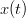$x(t)$ and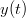$y(t)$.

### periodogram or pwelch

Calculates the power spectral density (PSD) function of signal$x(t)$. The periodogram may use several window functions as option. The pwelch will use the welch window.

### mscoherence

Calculates the coherence-squared spectrum function. If you apply an inverse Fourier transform (IFT), then you get the cross-correlation function in a very efficient way.